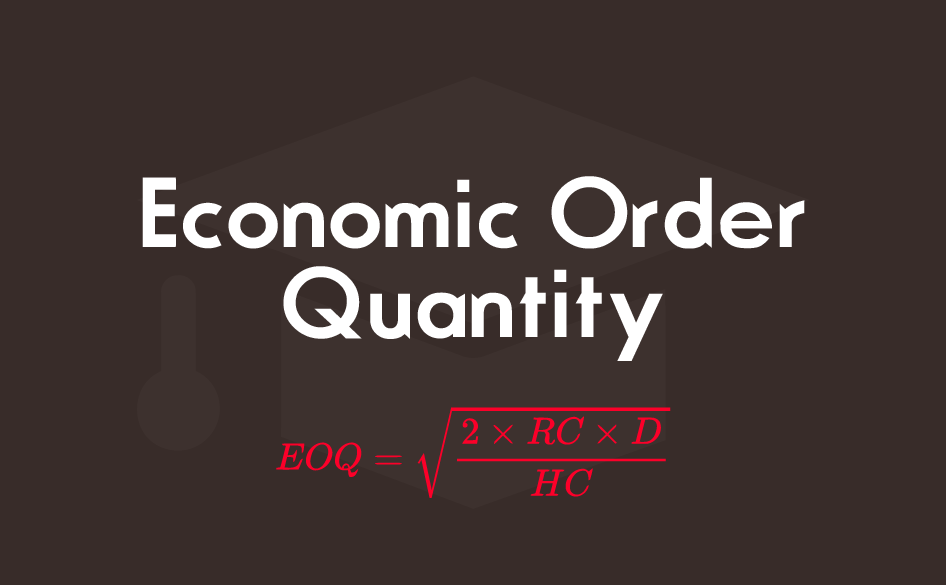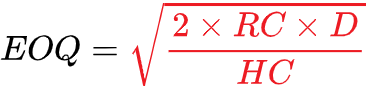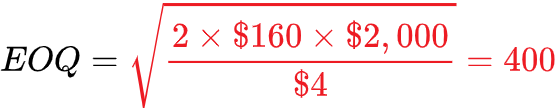# How much products to buy? | Inventory ManagementIf you want to minimize costs with your inventory logistics, you’ll need to figure out the optimal number of units you should purchase when the time arrives to replenish it.

In 1915, Harris of General Electric, developed a formula to help inventory managers and retailers determine how much product to buy.

That formula is called the economic order quantity (EOQ) and is the most important analysis of inventory control. It will help you operate in your supply chain logistics efficiently while keeping your costs down.

There are a number of assumptions with this formula:

• The demand is known and is constant over time
• All costs (reorder costs) are known and do not vary
• Delivery is made as soon as the order is placed

To calculate the economic order quantity (EOQ), assume:

D    = demand (the total number of units sold in a year)
RC = reorder cost (the cost of placing an order (includes equipment, quality checks, etc.)
HC = holding cost (the cost of holding the item per year (includes warehousing costs, insurance, etc.)Let’s see an example:

D = \$2,000 units sold a year
RC = \$160
HC = \$4 a unit a yearYour optimal order quantity is 400 units per order for that specific product.

If you want to know how many orders are expected for the year, you can divide the annualized demand with the results of the EOQ formula. This is called the period order quantity. Using our previous example, we should expect 5 orders per year:EOQ calculations might not consider all the factors but it’s perfect for reaching the optimum level of quantity and frequency of orders for a particular level of demand. It will help you save money, get better profits and analyze your inventory to keep it as efficient as possible.